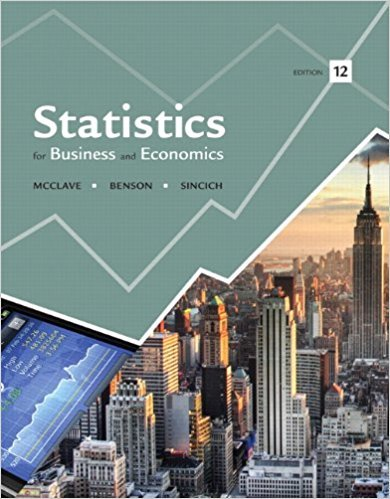×
Get Full Access to Statistics For Business And Economics - 12 Edition - Chapter 5 - Problem 1e
Get Full Access to Statistics For Business And Economics - 12 Edition - Chapter 5 - Problem 1e

×

# The probability distribution shown here describes aISBN: 9780321826237 51

## Solution for problem 1E Chapter 5

Statistics for Business and Economics | 12th Edition

• Textbook Solutions
• 2901 Step-by-step solutions solved by professors and subject experts
• Get 24/7 help from StudySoup virtual teaching assistantsStatistics for Business and Economics | 12th Edition

4 5 1 321 Reviews
23
3
Problem 1E

Problem 1E

The probability distribution shown here describes a population of measurements that can assume values of 0, 2, 4, and 6, each of which occurs with the same relative frequency:

 x 0 2 4 6 p(x) ¼ ¼ ¼ 1/4

a. List all the different samples of n = 2 measurements that can be selected from this population.

b. Calculate the mean of each different sample listed in part a.

c. If a sample of n = 2 measurements is randomly selected from the population, what is the probability that a specific sample will be selected?

d. Assume that a random sample of n = 2 measurements is selected from the population. List the different values of found in part b and find the probability of each. Then give the sampling distribution of the sample mean in tabular form.

e. Construct a probability histogram for the sampling distribution of .

Step-by-Step Solution:

Step 1 of 6:

Given the probability distribution describes a population of measurements that can assume values of 0, 2, 4 and 6 each of which occurs with the same relative frequency.

 x 0 2 4 6 p(x)Step 2 of 5

Step 3 of 5

##### ISBN: 9780321826237

Unlock Textbook Solution